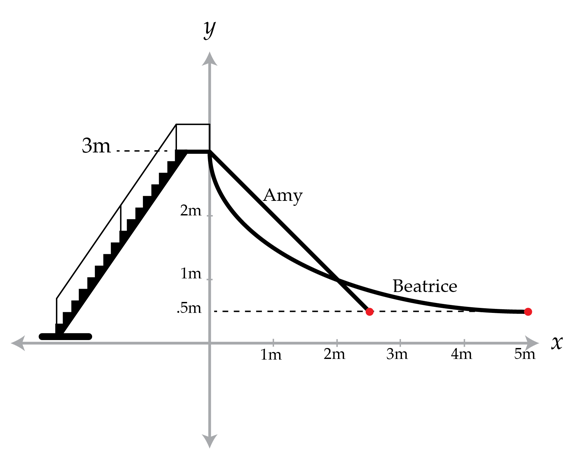Classical Mechanics

# Work-kinetic energy theorem

A $8.0\text{ kg}$ block is moving at $3.2\text{ m/s}.$ A net force of $10\text{ N}$ is constantly applied on the block in the direction of its movement, until it has moved $16\text{ m}.$ What is the approximate final velocity of the block?

A man is driving a car with mass $1.00 \times 10^3\text{ kg}$, at a speed of 35 m/s on a highway. Suddenly, he puts on the brakes to avoid an accident. After the brakes are applied, a constant kinetic friction force of magnitude $7.00 \times 10^3\text{ N}$ acts on the car. What is the minimum distance from the car ahead at which the brakes should be applied to avoid an accident?

Ignore air resistance.

Two identical twins, Amy and Beatrice, are on top of two different slides, one on each slide. Both slides start 3 m above the ground and end 0.5 m above the ground. Amy's slide is a straight, 45 degree slide with height given by $h=-x+3$ (so it starts at $x=0$ with a height of three meters and ends at $x=2.5$with a height of half a meter). Beatrice's slide is curved and the height is given by $h=3/(1+x)$ (so it starts at $x=0$ and ends at $x=5$). Both slides are frictionless.

If both twins start from rest at the top of their slides, what is the ratio of Amy's speed to Beatrice's speed at the bottom of the slides? i.e., what is $v_{Amy}/v_{Beatrice}$?The cannons on 19th century frigates were ponderous things. For example, on the USS Constitution, a frigate used in the War of 1812, the cannons were $3~\mbox{meters}$ long, had a mass of $3000~\mbox{kg}$, and could fire a $10 ~\mbox{kg}$ cannonball up to a kilometer away. Needless to say the recoil on the cannons was tremendous and a mechanism had to be devised to keep the cannons from careening across the deck. The system employed at the time consisted of rope and tackle, which basically caught the cannon. One wants to make sure that the ropes are strong enough, so one needs to know the magnitude of the forces necessary to stop the cannon. A cannon $4~\mbox{m}$ above the waterline fires a $10~\mbox{kg}$ cannonball horizontally that hits the water $500~\mbox{m}$ away. It recoils and is stopped by a rope braking mechanism, which exerts a constant force on the cannon once it starts to recoil. If the cannon recoils $1~\mbox{m}$, what is the force the rope braking mechanism exerts on the cannon in Newtons?

Details and assumptions

• You may neglect air resistance for the motion of the cannonball.
• The acceleration due to gravity is $-9.8~\mbox{m/s}^2$.

A man is driving his $1100\text{ kg}$ car at $36\text{ km/h}$ on a straight freeway. After accelerating for $30$ seconds, the car has a speed of $108\text{ km/h}.$ How much work did the engine do during the $30$ seconds?

Disregard the work lost by friction.

×